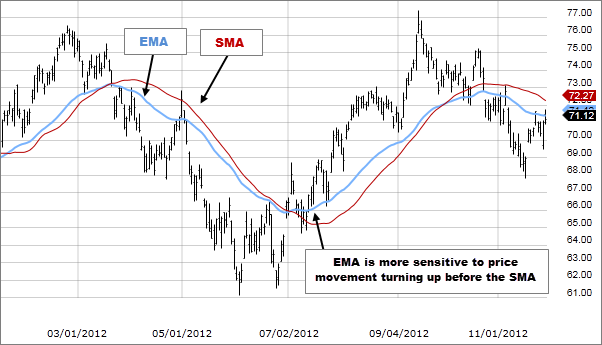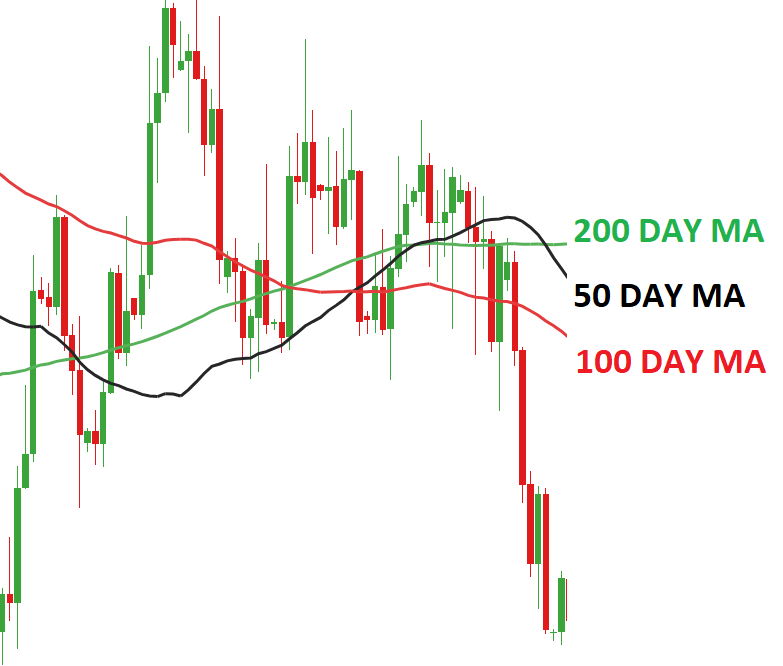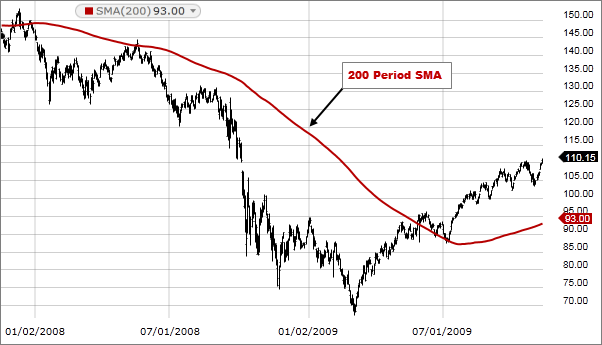# Exponential Moving Average vs Simple Moving Average# Exponential Moving Average vs Simple Moving Average

If you are just getting into the world of trading, then one of the most important things that you need to know about our moving averages. If you don’t know what a moving average is in comparison to stock market and Forex trading, then you are going to have a big problem making money. When it comes to technical analysis, moving averages are some of the most important things that you need to know about.

With that being said, there are two different types, and these include the simple moving average and the exponential moving average. Both provide you with the same type of information, but they are quite different and quite honestly one is better than the other. Today we are here to compare the exponential moving average to the simple moving average to see exactly what they are and what the differences between them are.## What is a Moving Average?

OK, so a moving average is a specific type of technical analysis indicator that is commonly used in a variety of trading markets, including the stock market, Forex, cryptocurrencies and more. Now, in terms of the four different types of indicators out there which include volume, trend, volatility and momentum indicators, this is actually a type of trend indicator.

In other words, a moving average can help you as a trader keep track of price trends for specific securities or assets.

If there is an upward trend in a moving average, it could signify an increase in the price or the momentum of a specific security and vice versa. To put it in simplest terms, a moving average will inform you of the trend of a specific security, or in other words, in which direction the price is moving, and it will also inform you of the momentum, to a certain degree.## Simple Moving Average: The Basics

The first type of MA that we want to look at is the simple moving average, which as you might be able to guess by the name of it, is the simpler of the two types of moving averages. To provide you with a basic definition, the SMA calculates the average of the price within a selected range.

In other words, it takes a specific amount of closing prices of a security or asset and then divides it by the number of periods in that range. The simple moving average to put it in simplest terms, is a technical indicator that can help determine if the price of an asset will continue to rise or if it will reverse.

In other words, they can tell you if there is a bull or bear trend on the horizon. This is a very simple yet effective trend indicator that Forex traders stock market traders and cryptocurrency trader used to determine when and where to place trades.### How to Calculate SMA

In case you are wondering how to calculate the SMAD, this is actually done quite easily. For instance, if you are calculating the SMA of a five day. Then you would take the closing prices of all five days an add them together and then divide them by five for those five days. Take a look at the extremely simple example below for a good idea of what the SMA calculation is all about.

\$8+\$9+10+11+12 = \$50

\$50/5 = 10

### Pros & Cons

Just as with any technical analysis indicator out there, that simple moving average does have a variety of benefits as well as some drawbacks too.

##### Pros
• The SMA offers a very smooth line that is not prone to website up and down in response to very slight and temporary price swings.
• The SMA is best used for longer periods of time.
##### Cons
• The primary drawback of the SMA is that it is very slow to respond to rapid price changes that occur at market reversal points.
• Because the SMA is quite slow to respond, it does not work well for short time frames.

## Exponential Moving Average

The next type of MA that we want to look at is the EMA, which as you can probably tell, is the more complicated type of moving average. The EMA or exponential moving average is another type of technical chart indicator that tracks the price of an investment such as a stock, commodity or even a currency pair over a specific period of time.

Just like the SMA, the EMA is also a trend indicator that can signify in which direction or what the trend of the prices, and to a certain degree it can also indicate the momentum of a security or asset’s price.

However, the big difference here is that the EMA is a type of weighted moving average or WMA, or in other words it gives more weight or importance to the most recent prices. Due to the fact that the exponential moving average puts the most weight or importance on the most recent prices, it is therefore ideal to use for short time frames.

With that being said, the exponential moving average is a bit more accurate and useful than the simple moving average. In terms of longer timeframes, the TMA can be used for 50 day, 100 day and 200 day periods. The typical time periods used for short term trading include the 12 day and 26 day EMAs.### How to Calculate EMA

Now, calculating the EMA is a bit difficult, so bare with us, as we will do our best to explain this to you in the simplest possible way. Yes, this is where things can get really confusing, but with a bit of practice, you should be able to master calculating an exponential moving average.

So, to calculate the EMA, you first need to obtain the SMA for a specific period of time. For instance, if you have a 20 day time period, you need to calculate the SMA for that period, and then, on the 21st day, you can use the SMA of the previous 20 days as the first EMA for yesterday.

You must then also calculate the weighting or the smoothing factor for the EMA, which is [2 / (number of observations) +1], which in this case would be [2/(20+1)] = 0.0952.

The final step of the calculation of the EMA is EMA = closing price x multiplier + EMA of previous day x (1 – multiplier). Yes, this is of course a bit complicated, but with just a little bit of practice, you should be able to master this calculation with relative ease. Just go to some price charts and give it a shot.

### Pros & Cons

Just like the simple moving average has benefits and drawbacks, so does the exponential moving average.

##### Pros
• Due to the fact that the most importance is given to the most recent prices, the exponential moving average responds very quickly to recent price changes. This can be very useful to intraday traders who are trading on very short time periods.
• The EMA is much better at calculating short term trend reversals and changes than the SMA.
##### Cons
• The disadvantage of the exponential moving average is that it’s fairly vulnerable to false signals and can be whipsawed back and forth.
• The exponential moving average is definitely not the best choice to go with for long term trading.

### FAQ

1. ##### What is the 20 EMA?

The 20 EMA is considered to be the best moving average to use for daily charts because the price follows it very accurately during a trend. If a price is above the 20 line, it can be considered a bullish trend, and if the price is below the 20 line, it can be considered a bearish trend.

1. ##### Why is 200 EMA Important?

The 200 EMA is considered to be very important for the identification of long term trend and can be easily applied to any security. It’s a great indicator to use to identify reversals.

1. ##### What is the 21 Day Exponential Moving Average?

The 21 day EMA places a whole 9.% of importance on the most recent price, whereas by comparison, the 100 day EMA places only 1.9% weight on the most recent price. Therefore, the 21 day EMA is excellent for identifying short term trends.

1. ##### What is the best EMA for Day Trading?

If you are engaging in short term trading, the best EMAs to use are the 8 day and 20 day EMAs, and for long term investors, the 50 day and 200 day EMAs work really well.

1. ##### Which EMA is Best for Forex?

Depending on what your needs are, the most commonly used EMAs in Forex include 5, 10, 12, 20, 26, 50, 100, and 200 day time frames.

1. ##### Which EMA is best for Intraday?

If you are intraday trading, then the 8 day and 20 day EMAs are usually best.

## Moving Averages – Final Thoughts

You should now know everything that there is to know about both the simple moving average and the exponential moving average. Now that you know the basics about them both, as well as what they are both ideal for, you can make an informed decision as to which one to use for your next trading adventure.

Remember folks, if you need help day trading, and what you need is a comprehensive education, particularly on Forex trading, then the best place to be is the Income Mentor Box Day Trading Academy. At this time, the IMB Academy is the most comprehensive, user friendly, effective, and affordable Forex trading school out there.

### CLICK BELOW TO JOIN INCOME MENTOR BOX AND START MAKING REAL PROFITS!